Full Text:<1722>

CLC number: P333

On-line Access: 2013-01-31

Revision Accepted: 2012-12-13

Crosschecked: 2013-01-23

Cited: 1

Clicked: 4189

Citations:  Bibtex RefMan EndNote GB/T7714

 Journal of Zhejiang University SCIENCE A 2013 Vol.14 No.2 P.110-119 http://doi.org/10.1631/jzus.A1200231

Modeling pollutant transport in overland flow over non-planar and non-homogenous infiltrating surfaces*

 Author(s):  Zhi-guo He1, Gokmen Tayfur2, Qi-hua Ran3, Hao-xuan Weng1 Affiliation(s):  1. Department of Ocean Science and Engineering, Zhejiang University, Hangzhou 310058, China; more Corresponding email(s):   ranqihua@zju.edu.cn Key Words:  Diffusion wave, Variation, Topography, Roughness, Infiltration, Pollutant, Modeling, Overland flow Share this article to： More <<< Previous Article|Next Article >>>

Zhi-guo He, Gokmen Tayfur, Qi-hua Ran, Hao-xuan Weng. Modeling pollutant transport in overland flow over non-planar and non-homogenous infiltrating surfaces[J]. Journal of Zhejiang University Science A, 2013, 14(2): 110-119.

@article{title="Modeling pollutant transport in overland flow over non-planar and non-homogenous infiltrating surfaces",
author="Zhi-guo He, Gokmen Tayfur, Qi-hua Ran, Hao-xuan Weng",
journal="Journal of Zhejiang University Science A",
volume="14",
number="2",
pages="110-119",
year="2013",
publisher="Zhejiang University Press & Springer",
doi="10.1631/jzus.A1200231"
}

%0 Journal Article
%T Modeling pollutant transport in overland flow over non-planar and non-homogenous infiltrating surfaces
%A Zhi-guo He
%A Gokmen Tayfur
%A Qi-hua Ran
%A Hao-xuan Weng
%J Journal of Zhejiang University SCIENCE A
%V 14
%N 2
%P 110-119
%@ 1673-565X
%D 2013
%I Zhejiang University Press & Springer
%DOI 10.1631/jzus.A1200231

TY - JOUR
T1 - Modeling pollutant transport in overland flow over non-planar and non-homogenous infiltrating surfaces
A1 - Zhi-guo He
A1 - Gokmen Tayfur
A1 - Qi-hua Ran
A1 - Hao-xuan Weng
J0 - Journal of Zhejiang University Science A
VL - 14
IS - 2
SP - 110
EP - 119
%@ 1673-565X
Y1 - 2013
PB - Zhejiang University Press & Springer
ER -
DOI - 10.1631/jzus.A1200231

Abstract:
pollutant transport in overland flow over surfaces with spatially varying microtopography, roughness, and infiltration was investigated using the diffusion wave equation and transport rate-based equation. The finite volume method in space and an implicit backward difference scheme in time were employed in the numerical solution of the 2D governing equations. The developed model was first tested against an analytical solution and an experimental study involving overland flow and the associated pollutant transport, subsequently a series of numerical tests were carried out. Non-point source pollution was investigated under spatially varying microtopography, roughness, and infiltration. The simulation results showed that microtopography and roughness were the dominant factors causing significant spatial variations in solute concentration. When the spatially varying microtopography was replaced by a smooth surface, the result was an overestimation of the solute rate at the outlet of the upland. On the other hand, when the spatially varying roughness was replaced by the average roughness and spatially varying infiltration rate by the average infiltration rate, the pollutant discharge at the outlet of the upland was not significantly affected. The numerical results further showed that one cannot ignore the spatial variations of slope and roughness when investigating the local pollutant concentration distribution.

## 1.  Introduction

Pollutant in overland flow is a major source of contamination in receiving water bodies and in groundwater due to infiltration. These pollutants arise mostly from surface applied agricultural chemicals, pesticides, and faecal bacteria (Monaghan and Smith, ). Solute transfer from soil to surface water due to overland flow may also decrease the efficiency of applied nutrients and chemicals.

Solute transfer from soil to overland flow and subsequent transport in surface flow were extensively studied both experimentally and numerically (Snyder and Woolhiser, ; Wallach et al., ; ; ; Payten and Sanders, ; Wallach and Van Genuchten, ; Wallach and Shabtai, ; Govindaraju, ; Garcia-Navarro and Playan, ; Yan and Kahawita, ; Walton et al., ; Abbasi et al., ; Deng et al., ; ; He et al., ; Ouyang et al., ). Most of the previous studies carried out were generally 1D physical experiments in laboratories and 1D solute transport mathematical modeling (Govindaraju, ; Garcia-Navarro and Playan, ; Yan and Kahawita, ; Walton et al., ; Wallach et al., ; Abbasi et al., ). 2D models have also been developed and tested against laboratory experiments and field investigations (VanderKwaak, ; Yan and Kahawita, ).

A difficulty that often arises in modeling pollutant transport in the rainfall-runoff process is the determination of the initial value of concentration in overland flow. Many models in previous studies often assume this value to be zero or equal to the initial concentration in soil surface for simplicity and convenience of both the numerical and analytical solutions of pollutant transport, which is not physically reasonable in the pollutant transport processes during rainstorms. In fact, a very high initial concentration caused by the first-rain-flush was observed, instead of the commonly assumed zero value. Deng et al. () developed a transport rate-based model for overland flow and solute transport instead of the commonly assumed zero value.

In the numerical modeling studies, natural surfaces, over which the transport takes place, are often simplified with smooth surfaces having constant average slope in one direction (in 1D case) or average constant slopes in two directions (in 2D case) (Wallach et al., ; ; Wallach et al., ; Govindaraju, ; Singh, ). Furthermore, the numerical studies most often assumed homogenous physical properties, thus employing a global roughness coefficient and constant infiltration parameters for the entire field surface (Wallach and Van Genuchten, ; Deng et al., ). In the case of planar surfaces with homogeneous properties, such assumptions might be plausible. However, one can rarely find such surfaces in nature. The effects of variability of topography, hydraulic properties, and vegetation density on soil erosion have been studied by modeling and measured data (Tayfur and Singh, ; Helmers et al., ; Helmers and Eisenhauer, ). It is our hypothesis that varying microtopography, roughness, and surface-cover properties of a field may play important roles in the transport of pollutants in overland flow. These characteristics may alter the spatial and temporal variation of pollutants in the field, and into the soil column by infiltration. In addition, these varying characteristics may alter the magnitude of pollutant rates from agricultural fields into the adjacent receiving water bodies. Thus, any modeling studies ignoring the effects of non-planar and non-homogeneous physical effects of the field on the pollutant transport may lead to misleading results. To the knowledge of the authors, so far, there has been no study conducted to investigate effects of varying microtopography, surface-cover, and roughness on pollutant transport in overland flows. Thus, the objective of this study is to numerically investigate effects of varying microtopography, roughness, and infiltration on pollutant transport in overland flows.

## 2.  Mathematical model

### 2.1.  2D overland flow model

The depth-averaged 2D governing equation under the diffusion wave approximation for overland flow can be expressed as (Govindaraju, ) , , where h is the water flow depth; R is the rainfall rate; I is the infiltration rate; u and v are the overland flow velocities in x and y directions, respectively; S fx and S fy are the friction (energy) slopes in x and y directions, respectively; nx and ny are the Manning roughness coefficients in x and y directions, respectively, if anisotropy in roughness is considered; and .

The diffusion wave model has been shown to provide satisfactory results in most free surface flow applications (Akan and Yen, ; Tayfur et al., ; Tayfur and Singh, ). Infiltration rate in this study was modeled by assuming a constant infiltration rate.

Solution of Eq. (1) requires initial condition and conditions at the upstream and downstream boundaries. The upstream boundary condition was expressed by the zero flow depth and the downstream boundary condition by the zero flow depth-gradient, which have been commonly used in (Tayfur et al., ; Tayfur and Singh, ). For the initial condition, a very thin water layer of 1×10−6 mm for flow depth was assumed to avoid the singularity problem in the numerical solution of Eq. (1).

### 2.2.  2D pollutant transport model

During rainstorms, a pollutant in the uppermost layer of the soil is often released to the overland flow. Based on few observations, this study assumes that the solute in this surface active layer are uniformly distributed and the exchange rate of the solute between the active layer and overland flow are proportional to the difference between the concentrations in soil and water volume (Deng et al., ). Generally, pollutant transport in overland flow can be described by the advection-dispersion equation (ADE) (Govindaraju, ). However, for overland solute transport, the diffusion/dispersion term can be negligible as compared to other terms (Abbott and Refsgaard, ; Wallach et al., ). Thus, pollutant transport equation in 2D can be expressed as , where c is the pollutant concentration in overland flow; c s represents the solute concentration in the active surface layer with a thickness of D m=εh, with h being the overland flow depth and ε being a dimensionless coefficient; R is the exchange rate of solute between the active surface layer and the overlying flow; J c is the flux across the water surface per unit surface area; and q co represents other source or sink terms.

To avoid the uncertainty in actual initial high level concentration conditions, this study employs the definition of the transport rate of solute, which is the product of surface runoff and solute concentration. By replacing c by c=C/Q f and c s by c s=C s/Q f, and splitting each differential term of Ch/Q f into two terms, i.e., Ch and 1/Q f, and then multiplying both sides of the equation by Q f, Eq. (3) yields the following rate-based solute transport equation: , where C is the transport rate of solute by overland flow, C s is the transport rate of solute from the soil to the overland flow, E=εR, and .

For a steady and net rainfall flow, in Eq. (4) approaches zero. Decline of the solute transfer rate in the active surface layer caused by the transfer of solutes from soil to the overlying runoff can be assumed to follow an exponential law with a time constant μ as C s=C 0exp(−μt) (Deng et al., ).

The initial condition of Eq. (4) is then given as C(x,0)=0 and C s(x,0)=C 0(x), in which C 0 is the initial transport rate of solute from the soil to the overland flow. The upstream boundary condition was taken as the zero solute transport rate and the downstream boundary condition as the zero gradient of solute transport rate. Eq. (4) can be solved as long as the parameters, E, μ, and C 0 are determined.

## 3.  Numerical scheme

The governing Eqs. (1) and (4) can be rewritten in the following general form: , where Φ represents h and Ch for the surface flow equation and pollutant transport equation, respectively; and S cΦ includes all source terms in the equation for Φ.

The equations governing surface flow and pollutant transport are discretised using the finite volume method in space and an implicit scheme in time. In the rectangular 2D control volume, the discretised flow and pollutant transport equations are expressed as .

Picard iterative technique was applied to handle the nonlinear equations. The discretised equations were solved by the strongly implicit procedure (SIP) of Stone (). Accurate numerical solution and mass balance are reached when the convergence criteria of both the Picard iteration and SIP solution loops are satisfied at each time step.

In the discretized surface equation, variables at the interface of control volume are evaluated by an upstream scheme, which ensures the solution monotonicity. The fluxes in Eq. (6) are functions of the water depth and velocities and are determined with the operator ψ. The operator ψ, which is the nonlinear function of the surface roughness and the magnitude of the water potential (friction slope), is evaluated as a cell parameter at the previous iteration level as , where εm is a small number (e.g., 10−8 to 10−10) that, in the event of a zero fluid potential gradient, avoids a division by zero in Eq. (6) by enforcing a minimum value of Ψ (VanderKwaak, ). The pollutant transport is then calculated after flow computations.

## 4.  Model verification

### 4.1.  Case 1

The present pollutant transport model is first tested against an analytical solution for transport of dissolved chemicals in overland flow, given by Rivlin and Wallach (). In their mathematical model, the chemical flux, R ch, across the soil surface was determined as R ch=k ch(C soilC water), with k ch being a mass transfer coefficient, and C soil and C water being the concentrations of chemical at the soil surface and the runoff water, respectively (Rivlin and Wallach, ; Wallach et al., ). The analytical solution of water depth and chemical concentration at the outlet of a 100 m long uniform slope was calculated for a rainfall rate of 1.6 cm/h, a duration of 0.25 h, an infiltration rate of 0.3 cm/h, and k ch=0.9 cm/h. Fig. 1 shows the comparison of calculated hydrograph and concentration, respectively. A good agreement between the numerical results and analytic solutions for both overland flow depth and chemical concentration at the slope outlet was found with the maximum error less than 8%.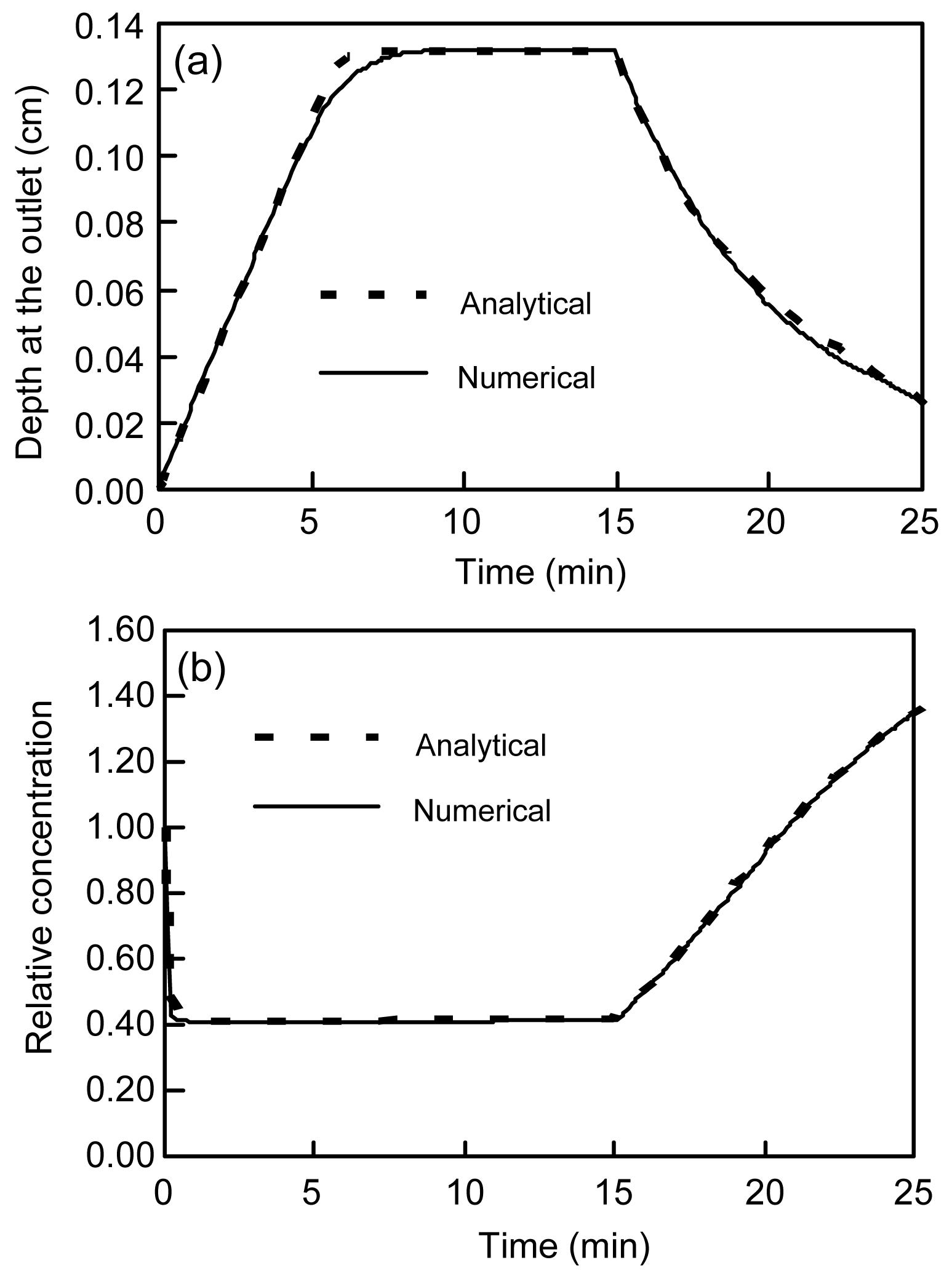Fig.1
Simulated flow depth and analytical solution (a); simulated relative concentration and analytical solution (b)

### 4.2.  Case 2

The developed model was further tested using an experimental study that investigated overland flow and associated pollutant transport over the soil surface during rainstorms (Deng et al., ). The experiment was carried out using a rainfall simulator and a soil flume with a dimension of 3.0 m×0.3 m×0.12 m (length×width×height). Prior to running the experiments with a constant rainfall rate, the soil was wet to field capacity and 0.2 kg of granular salt with a mean diameter of 0.4 mm was applied uniformly onto the soil surface. The saturated water content was 39%, and the initial water content was 20%. During the experiments, surface runoff with solute was collected at the end of the flume. The discharge, salt concentration, and solute transport rate were then measured. The experimental conditions can be found in Table 1. The characteristics of soil and rainfall simulator are given in (de Lima et al., ) and the details of experiment can be obtained from Deng et al. ().

#### Table 1

Experimental conditions
 Flume slope Rainfall intensity (mm/h) Rainfall excess (mm/h) Infiltration capacity (mm/h) Rainfall duration (min) Flow discharge (L/s) Amount of pollutant (g/m2) Hydraulic conductivity (m/s) 10% 263.2 215.6 47.6 17.3 0.055 222.2 5.7×10−5

To simulate the experiment, the developed model employed a mesh size of 22×22. The parameters used in the simulation, which were calibrated by Deng et al. (), are listed in Table 2. Fig. 2 shows the comparisons of measured and computed hydrographs and pollutographs. Although the measured discharge varied significantly as shown in Fig. 2a, a relative constant value of 0.055 L/s was obtained. The averaged relative error of measured and simulated discharges was about 5.4%. Figs. 2b and 2c compare the observed and simulated solute transport rates and concentrations, respectively. Both figures show that the simulated results using the developed model are in a good agreement with the experimental data.

#### Table 2

Parameters in the simulation (Deng et al., )
 n E (1/s) μ (1/s) C 0 (g/s) 0.025 0.04 0.022 3.68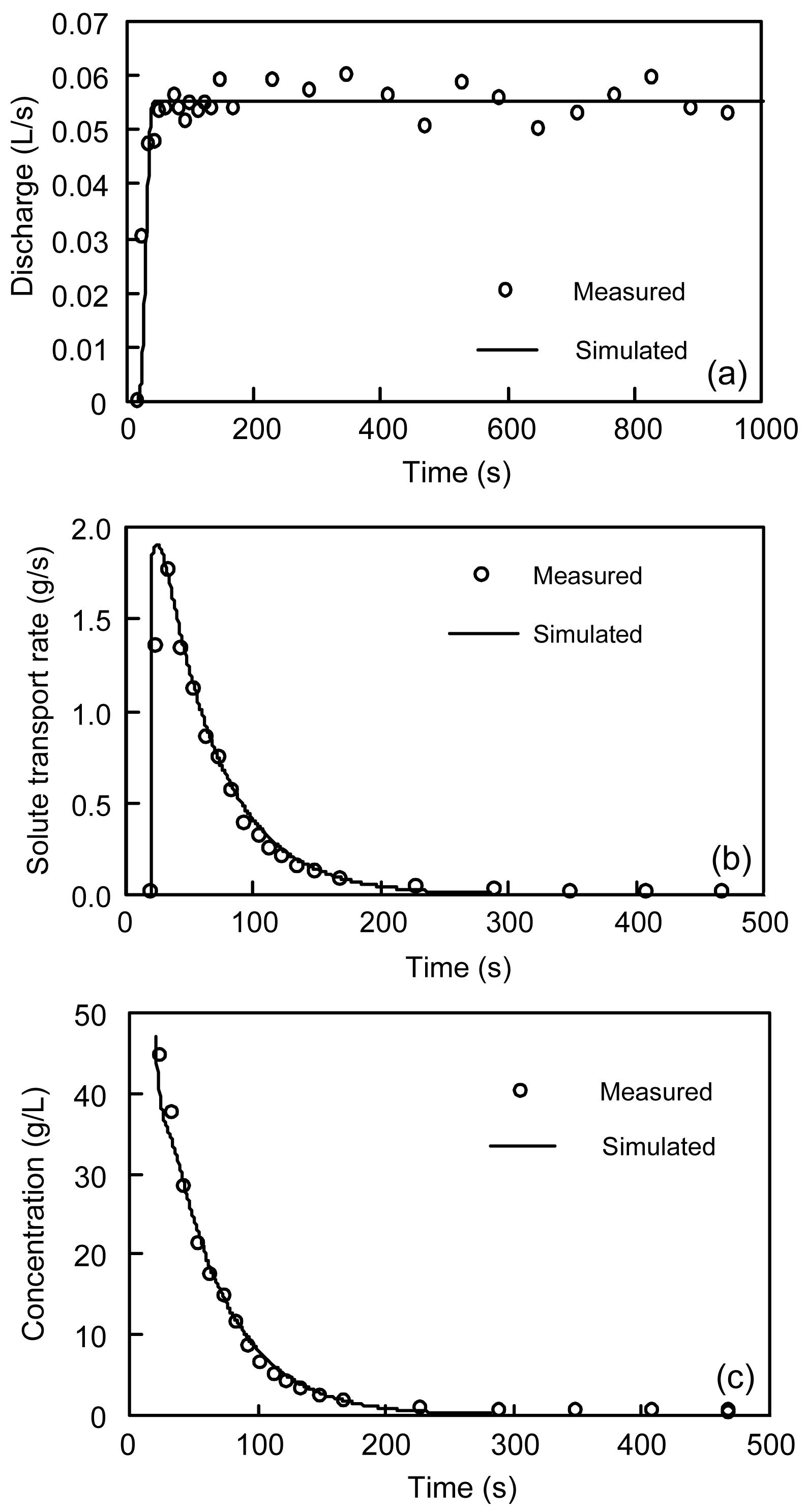Fig.2
Comparison between measured and simulated hydrographs (a), solute transport rates (b) and pollutographs (c)

## 5.  Numerical investigation

To investigate the effects of spatially varying microtopographic surface, roughness, and infiltration rate with their corresponding average values on the pollutant transport dynamics, a set of hypothetical data for these parameters variably distributed over soil flume was generated based on the parameters in Case 2. However, the width of the simulation domain in this section is extended from 0.3 m to 3 m to consider the 2D behavior of pollutant transport. The model simulated the overland flow and pollutant transport over 3 m by 3 m soil flume with a constant slope of 10%, a Manning’s roughness coefficient of 0.025, and a constant infiltration rate of 47.6 mm/h as a basis case.

The hypothetical spatially varying topographic surface was generated over 3 m by 3 m plane according to the random distribution function with a mean of 0.10 and a standard deviation of 0.029, as shown in Fig. 3a. The range of the generated slope data was 0.05–0.15.Fig.3
Spatial distribution of microtopographic surface (a), surface roughness (b) and infiltration rate (c)

The hypothetical spatially varying surface roughness was generated over 3 m by 3 m plane according to the random distribution function with a mean of 0.025 and a standard deviation of 0.0089, as shown in Fig. 3b. The range of the generated data was 0.01–0.04, corresponding to bare sand (n=0.010–0.016), gravel surface (n=0.012 to 0.030), bare clay-loam soil (n=0.012 to 0.033), soil with grass (n=0.03 to 0.05), which is physically sound.

The hypothetical spatially varying infiltration rate was generated over 3 m by 3 m plane according to the random distribution function with a mean of 47.6 mm/h and a standard deviation of 21.33 mm/h, as shown in Fig. 3c. The range of the generated data was 10–85 mm/h, corresponding to loam (I=3.4 mm/h), silt loam (I=6.5 mm/h), sandy loam (I=11 mm/h), loamy sand (I=30 mm/h), and fine sand (I=100 mm/h), which is physically sound.

Note that one may generate many different random fields of microtopographic surface, surface roughness, and infiltration rate with the same mean and standard deviation. However, to have an insight into the effects of employing varying parameters (as opposed to constant average values) on the pollutant concentration profile, one may employ only one realization, as is discussed in this study.

Fig. 4 shows velocity, streamline, water depth, solute transport rate, and concentration distributions under the spatially varying microtopographic surface, at a time equal to 128 s when runoff reaches steady. As shown in Figs. 4b and 4c, the concentration and pollutant transport rate have non-smooth profiles, clearly reflecting the impact of the spatially varying slopes which influence the flow depth, velocity, and flow path, as shown in Fig. 4a. Fig. 5 gives solute transport rates and pollutographs at the outlet computed for the cases considering constant and variable slope surface. The transport rates use three sets of variable slopes different in space but with the same mean and standard deviation as shown in Fig. 5a. The variable slopes do not significantly influence the results when they have the same mean and standard deviation. The employment of average constant slopes overestimated the pollutant rate at the outlet by about 9%, while there was no significant influence on concentration.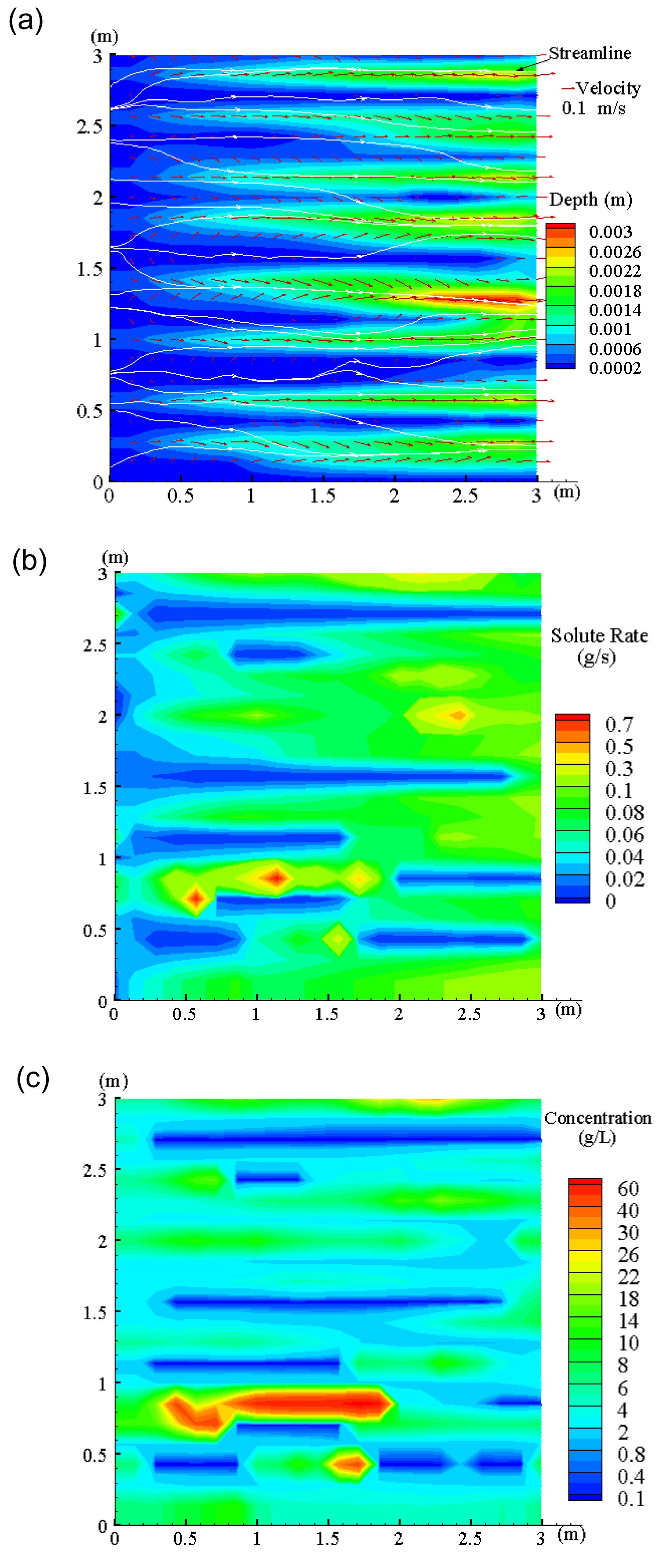Fig.4
Flow field (a), solute rate (b) and concentration (c) under the spatially varying microtopographic surface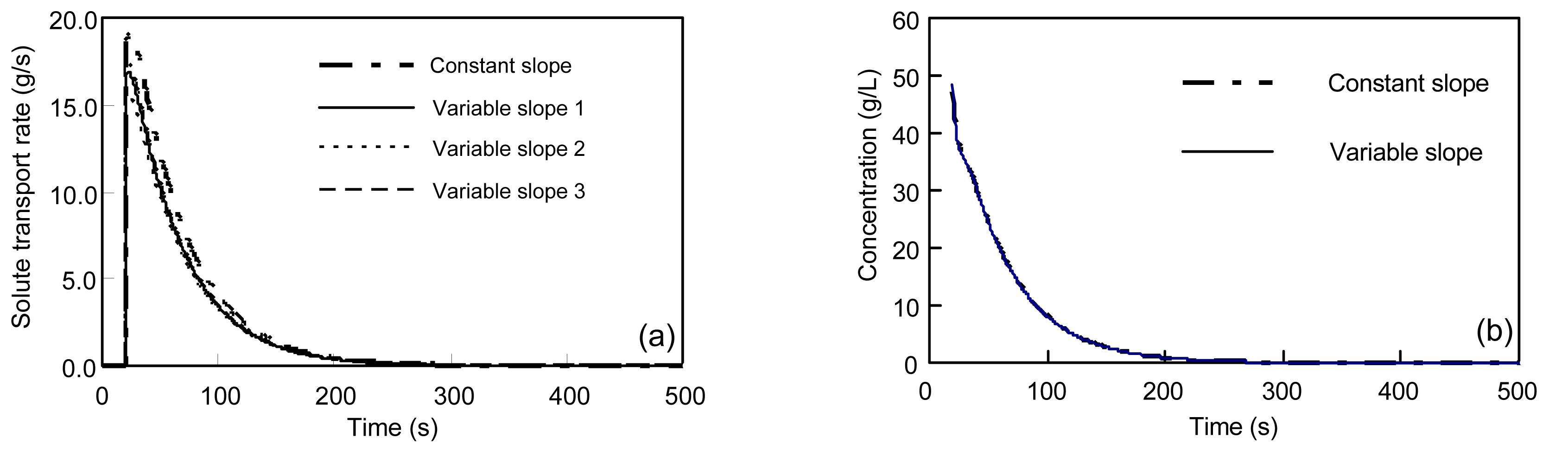Fig.5
Comparison of pollutant transport rate (a) and concentration (b) under constant (10%) and variable surface slopes (mean slope=0.1 and standard deviation=0.029)

Fig. 6 shows water depth, concentration, and solute transport rate under the spatially varying surface roughness, at a time equal to 128 s when runoff reaches steady state. Although both concentration and transport rate profiles are impacted by the variable surface roughness and not smooth, the variable roughness has less impact on pollutant transport than varying slopes. This is because the variation of roughness has less influences on the flow depth, velocity and flow path in comparison with the variation of topography. The concentrations in the upstream are generally higher than those in the downstream, and the solute transport rates show the reverse trend. Fig. 7 compares the concentrations and pollutant transport rates at the outlet under constant and variable surface roughness. The transport rates use three sets of variable roughness different in space but with the same mean and standard deviation (Fig. 7a). The results indicate that the pollutographs computed on the basis of the uniform roughness surface well approximate those obtained from the spatially variable roughness surface.Fig.6
Water depth (a), concentration (b) and solute transport rate (c) under the spatially varying surface roughness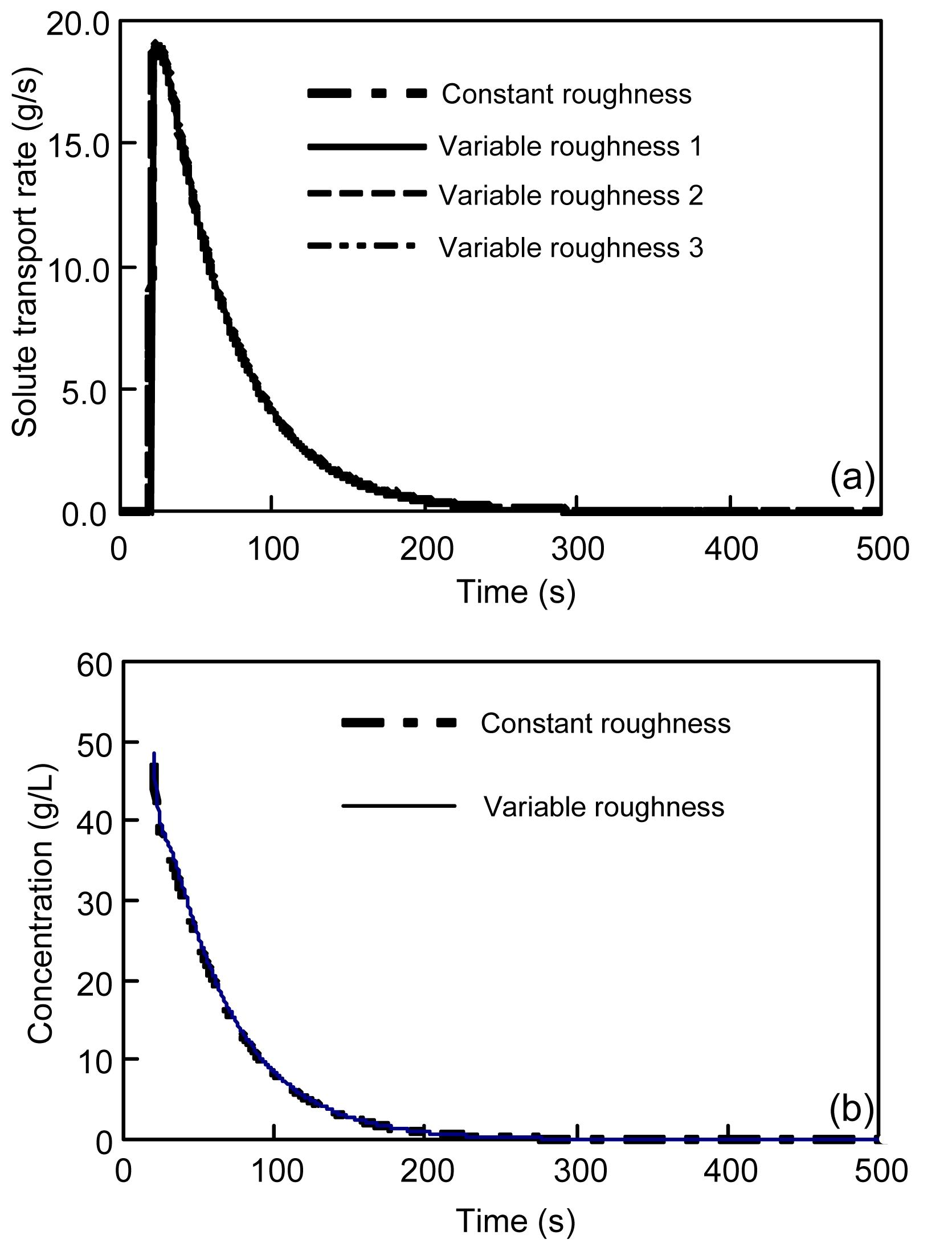Fig.7
Comparison of pollutant transport rate (a) and concentration (b) under constant (n=0.025) and variable surface roughnesses (mean=0.025 and standard deviation 0.0089)

Fig. 8 shows water depth, concentration, and solute transport rate under the spatially varying infiltration rate, at a time equal to 128 s when runoff reaches steady state. Similar to the variable roughness, the variable infiltration rate has less impact on both concentration and transport rate profiles, as opposed to the variable slope case. Fig. 9 compares the concentrations and pollutant transport rates at the outlet under constant and variable infiltration rates. The transport rates use three sets of variable infiltration rate different in space but with the same mean and standard deviation (Fig. 9a). The results show insignificant difference between the cases with different infiltration rates.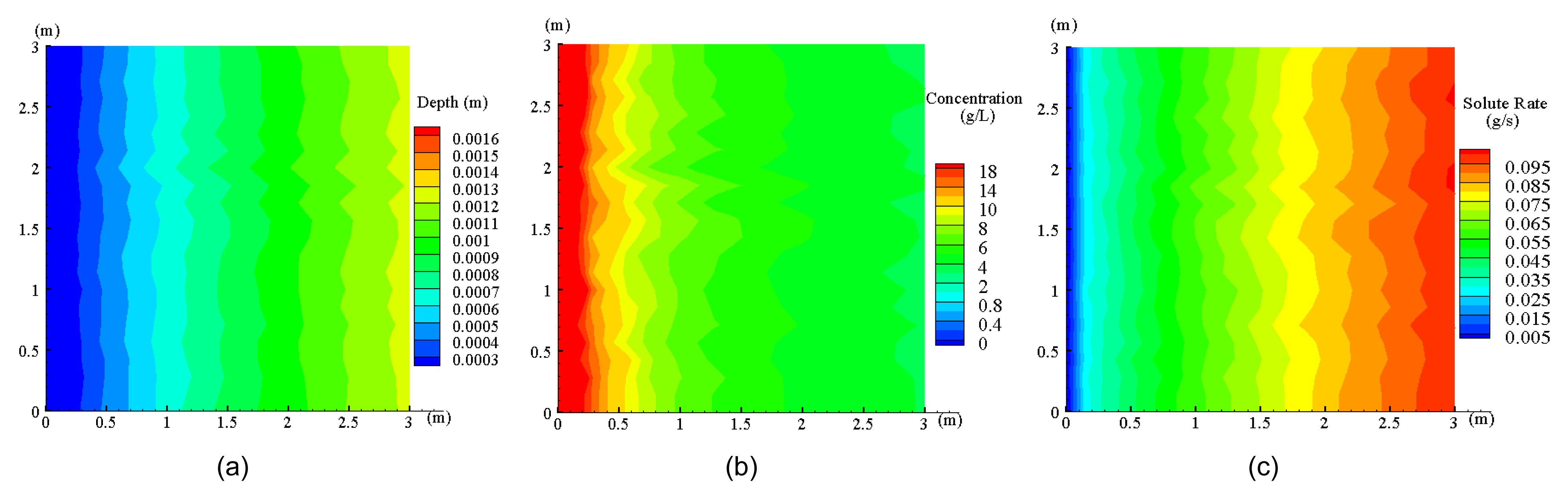Fig.8
Water depth (a), concentration (b) and solute transport rate (c) under the spatially varying infiltration rateFig.9
Comparison of solute transport rate (a) and concentration (b) under constant (I=47.6 mm/h) and variable infiltration rates (mean=47.6 mm/h and standard deviation=21.33 mm/h)

## 6.  Conclusions

A 2D pollutant transport rate-based model combined with the diffusive wave surface flow was developed for simulating overland flow and the associated pollutant transport over surfaces with spatially varying microtopography, roughness, and infiltration. The developed model was tested using a set of analytical solution and laboratory data. The satisfactory simulations of the analytical and experimental data verify the accuracy of the model. The effects of varying slope, roughness, and infiltration on pollutant transport during rainstorms were then numerically investigated by the model. Based on the numerical investigation in this paper, the following conclusions can be drawn:

1. The effects of varying slopes on the flow dynamics, pollutant rates and concentrations are very pronounced. Pollutant load could be overestimated by about 10% if an actual spatially varying microtopography is replaced by constant average slopes. Surfaces are rarely smooth in nature, and the constant-slope assumption is far from reality.

2. The effect of varying roughness on pollutant transport rate and/or concentration at the outlet is relatively negligible, and therefore the use of constant average roughness to represent varying roughness is justified. However, as far as local concentration measures are concerned, the varying roughness should not be replaced by average roughness.

3. Spatially varying infiltration could be represented by spatially averaged infiltration without undue loss of accuracy in estimating pollutant load at the outlet of upland.

* Project supported by the National Natural Science Foundation of China (No. 51009120), the Research Fund for the Doctoral Program of Higher Education of China (No. 20090101120065), the State Key Laboratory of Soil Erosion and Dryland Farming on the Loess Plateau of China (No. 10501-243)

## ReferencesAbbasi, F., Simunek, J., Van Genuchten, M.T., Feyen, J., Adamsen, F.J., Hunsaker, D.J., Strelkoff, T.S., Shouse, P., 2003. Overland water flow and solute transport: model development and filed data analysis. Journal of Irrigation and Drainage Engineering, 129(2):71-81.Abbott, M.B., Refsgaard, J.C., 1996.  Distributed Hydrological Modeling. Kluwer Acedemic Publisher,Dordrecht, The Netherland :Akan, A.O., Yen, B.C., 1981. Mathematical model of shallow water flow over porous media. Journal of Hydraulic Division, 107(4):479-494.de Lima, J.L.M.P., Singh, V.P., de Lima, M.I.P., 2003. The influence of storm movement on water erosion: storm direction and velocity effects. Catena, 52(1):39-56.Deng, Z.Q., Lima, J.L.M.P., Singh, V.P., 2005. Fractional kinetic model for first flush of stormwater pollutants. Journal of Environmental Engineering, 131(2):232-241.Deng, Z.Q., Lima, J.L.M.P., Singh, V.P., 2005. Transport rate-based model for overland flow and solute transport: parameter estimation and process simulation. Journal of Hydrology, 315(1-4):220-235.Garcia-Navarro, P., Playan, E., 2000. Solute transport modeling in overland flow applied to fertigation. Journal of Irrigation and Drainage Engineering, 126(1):33-40.Govindaraju, R.S., 1996. Modeling overland flow contamination by chemicals mixed in shallow soil horizons under variable source area hydrology. Water Resources Research, 32(3):753-758.He, Z., Wu, W., Wang, S.S.Y., 2009. An integrated 2D surface and 3D subsurface contaminant transport model considering soil erosion and sorption. Journal of Hydraulic Engineering, 135(12):1028-1040.Helmers, M.J., Eisenhauer, D.E., 2006. Overland flow modeling in a vegetative filter considering non-planar topography and spatial variability of soil hydraulic properties and vegetation density. Journal of Hydrology, 328(1-2):267-282.Helmers, M.J., Eisenhauer, D.E., Franti, T.G., Dosskey, M.G., 2005. Modeling sediment trapping in a vegetative filter accounting for converging overland flow. Transactions of the ASAE, 48(2):541-555.Monaghan, R.M., Smith, L.C., 2012. Contaminant losses in overland flow from dairy farm laneways in southern New Zealand. Agriculture, Ecosystems & Environment, 159:170-175.Ouyang, W., Guo, B., Hao, F., Huang, H., Li, J., Gong, Y., 2012. Modeling urban storm rainfall runoff from diverse underlying surfaces and application for control design in Beijing. Journal of Environmental Management, 113:467-473.Payten, R.L., Sanders, G., 1990. Mixing in overland flow during rainfall. Journal of Environmental Engineering, 116(4):764-784.Rivlin, J., Wallach, R., 1995. An analytical solution for the lateral transport of dissolved chemicals in overland flow. Water Resources Research, 31(4):1031-1040.Singh, V.P., 2002. Kinematic wave solution for pollutant transport over an infiltrating plane with finite-period mixing and mixing zone. Hydrological Processes, 16(12):2441-2477.Snyder, I.K., Woolhiser, D.D., 1985. Effects of infiltration on chemical transport onto overland flow. Transactions of. ASAE, 28:1450-1457.Stone, H.L., 1968. Iterative solution of implicit approximation of multidimensional partial differential equations. SIAM Journal of Numerical Analysis, 5:530-558.Tayfur, G., Singh, V.P., 2004. Numerical model for sediment transport over non-planar, non-homogeneous surfaces. Journal of Hydrologic Engineering, 9(1):35-41.Tayfur, G., Kavvas, M.L., Govindaraju, R.S., Storm, D.E., 1993. Applicability of St. Venant equations for two dimensional overland flows over rough infiltrating surfaces. Journal of Hydraulic Engineering, 119(1):51-63.VanderKwaak, J.E., 1999.  Numerical Simulation of Flow and Chemical Transport in Integrated Surface-Subsurface Hydrologic Systems. PhD Thesis, University of Waterloo,Waterloo, Ont, Canada :Wallach, R., Van Genuchten, M.T., 1990. A physically based model for predicting solute transfer from soil to rainfall-induced runoff. Water Resources Research, 26(9):2119-2126.Wallach, R., Shabtai, R., 1993. Surface runoff contamination by chemicals initially incorporated below the soil surface. Water Resources Research, 29(3):697-704.Wallach, R., Jury, W.A., Spencer, W.F., 1988. Transfer of chemicals from soil solution to surface runoff: a diffusion-based soil model. Soil Science Society of America Journal, 52(3):612-618.Wallach, R., Jury, W.A., Spencer, W.F., 1989. The concept of convective mass transfer for prediction of surface-runoff pollution by soil surface applied chemicals. Transactions on ASAE, 2(3):906-912.Wallach, R., Grigorin, G., Rivlin, J., 2001. A comprehensive mathematical model for transport of soil-dissolved chemicals by overland flows. Journal of Hydrology, 247(1-2):85-99.Walton, R.S., Volker, R.E., Bristow, K.L., Smettem, K.R.J., 2000. Experimental examination of solute transport by surface runoff from low-angle slopes. Journal of Hydrology, 233:19-36.Yan, M., Kahawita, R., 2000. Modeling the fate of pollutant in overland flow. Water Research, 34(13):3335-3344.Yan, M., Kahawita, R., 2007. Simulating the evolution of non-point source pollutants in a shallow water environment. Chemosphere, 67(5):879-885.